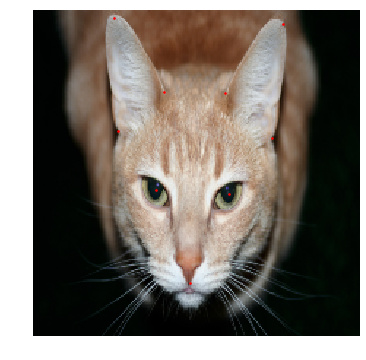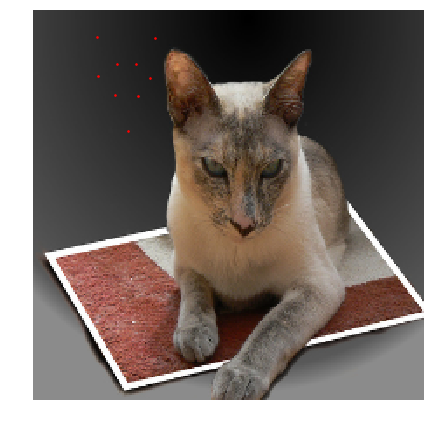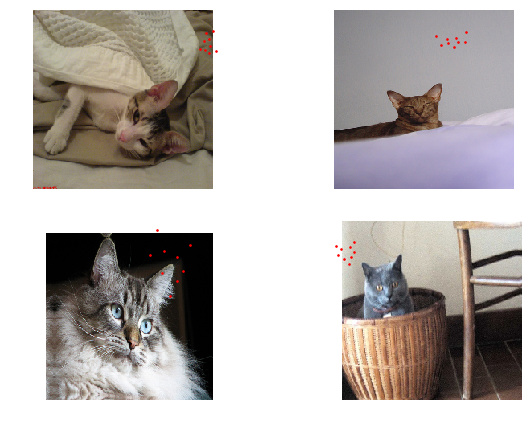# Landmarks missing the target after databunch creation

Hello,

I’m working on a regression problem using a cat dataset from kaggle. It is basically pictures of cats with landmarks of its facial features.

Anyway, the images comes in different sizes and I have to resize them for the datablock api to read the images without warnings. But first I need to rescaled the points with this small function:

``````from fastai.vision import *
import os
data_path = os.path.join(os.getcwd(), "data")

def get_labels(file):
points = (points_df.values[1:-1]).reshape((-1, 2))
points[:, [0, 1]] = points[:, [1, 0]]

old_size = open_image(os.path.join(file)).size
points_ratio =  np.array([224,224]) / np.array(old_size)

points = Tensor(points * points_ratio)

return Tensor(points)
``````

And I can plot a single image like this:

``````img_path = os.path.join(data_path, "CAT_01", "00000256_006.jpg")
img = open_image(img_path).resize(224)
points = ImagePoints(FlowField(img.size, get_labels(img_path)))
img.show(y=points, figsize=(9, 6))
``````However, when I create a databunch using the datablock API, and try to show the images using `show_batch`, the points seems way off:

``````data = (PointsItemList.from_folder(data_path, extensions=".jpg")
.split_by_rand_pct() # Later split by folder
.label_from_func(get_labels)
.transform(get_transforms(), size=(224, 224))
.databunch().normalize(imagenet_stats)
)

data.show_batch(1, figsize=(9, 6))
``````Am I missing something?

Thanks

You forgot to pass `tfm_y=True` in your call to transform@sgugger thanks for the help, but unfortunately that did not seem to have worked. This is what I’m running now:

``````data = (PointsItemList.from_folder(data_path, extensions=".jpg")
.split_by_rand_pct()
.label_from_func(get_labels)
.transform(get_transforms(), tfm_y=True, size=(224, 224), remove_out=False)
.databunch().normalize(imagenet_stats)
)
``````

And this the results inside show batch (sorry for the differents cats, can’t seem to find the same one).

``````data.show_batch(2, figsize=(9, 6))
``````You shouldn’t do the resizing in `get_labels`: your example work before you resize the image first, the create your points with that new size ( and have scaled them accordingly in your `get_labels` function)

BUT

What’s happening in the pipeline is that your points are created at the same time the original image is opened, with the old size of the image as a referent. So you do a first scaling in `get_labels` then the fastai library does another scaling during the tranforms call (that resize to 224). That’s why you have this problem.

Thanks a lot @sgugger!

It seems so obvious I don’t know how this went over my head.
I also rewatched part of the third lesson and there Jeremy explains about using `tfm_y`, makes a lot of sense.

Again thank you for your time.